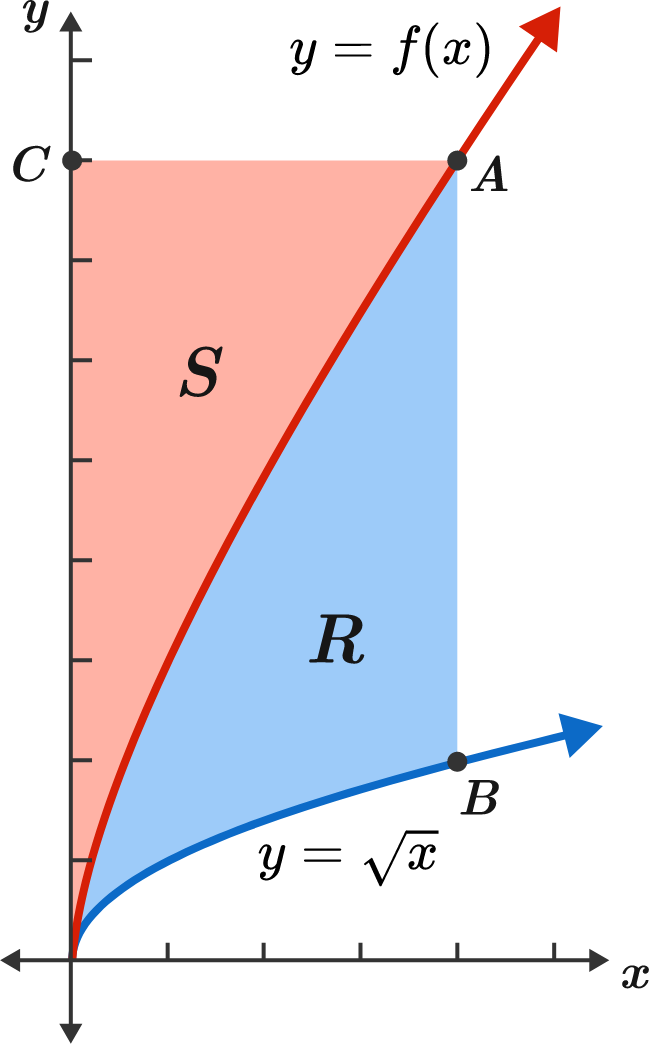# Area under the curves, can you make a right choice?

Calculus Level 2

Take a point $A$ on the graph of $y=f(x),$ and let $B$ be a point on the graph of $y=\sqrt{x}$ such that $AB$ is parallel to the $y$-axis. Call the area bounded by these two curves and the segment $AB$ as $R.$

Now, let $C$ be a point on the $y$-axis such that $AC$ is parallel to the $x$-axis. Call the area bounded by the curve $y=f(x),$ the $y$-axis, and the segment $AC$ as $S.$

Given that the function $f$ is continuous and the areas $R$ and $S$ are equal, which of the following statements is true?×